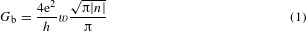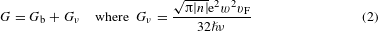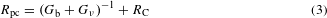## Main

Graphene hosts a high-quality electron system with weak phonon coupling20,21 such that e–e collisions can become the dominant scattering process at elevated temperatures, T. In addition, the electronic structure of graphene inhibits Umklapp processes15, which ensures that e–e scattering is momentum conserving. These features lead to a fluid-like behaviour of charge carriers, with the momentum taking on the role of a collective variable that governs local equilibrium. Previous studies of the electron hydrodynamics in graphene were carried out using the vicinity geometry and Hall bar devices of a uniform width. Anomalous (negative) voltages were observed, indicating a highly viscous flow, more viscous than that of honey12,22,23. In this report, we employ a narrow constriction geometry (Fig. 1a) which offers unique insight into the behaviour of viscous electron fluids. In particular, the hydrodynamic conductance through such constrictions becomes ‘superballistic’, exceeding the fundamental upper bound allowed in the ballistic limit, which is given by the Sharvin formula16,17. This is in agreement with theoretical predictions18,19 and is attributed to a peculiar behaviour of viscous flows that self-organize into streams with different velocities, with ‘sheaths’ of a slow-moving fluid near the constriction edges (Fig. 1b). The cooperative behaviour helps charge carriers to circumnavigate the edges, the only place where the electron flow can lose its net momentum. The physics behind is fairly similar to that involved in the transition from the Knudsen to Poiseuille regimes, well understood in gas dynamics, where the hydrodynamic pressure can rapidly drop upon increasing the gas density and the rate of collisions between molecules24.Figure 1: Electron flow through graphene constrictions.

Our devices are made of monolayer graphene encapsulated between hexagonal boron-nitride crystals, as described in Supplementary Section 1. The device design resembles a multi-terminal Hall bar, endowed with constrictions positioned between adjacent voltage probes (Fig. 1c). Below we refer to them as (classical) point contacts (PCs). Five such Hall bars were investigated, each having PCs of various widths w and a reference region without a constriction. The latter allowed standard characterization of graphene, including measurements of its longitudinal resistivity ρxx. All our devices exhibited mobilities exceeding 10 m2 V−1 s−1 at liquid-helium T, which translates into a mean free path exceeding 1 μm with respect to momentum-non-conserving collisions (Supplementary Section 2).

Examples of the measured PC conductance Gpc at 2 K are given in Fig. 1d. In the low-T regime, all scattering lengths exceed w and transport is ballistic, which allows Gpc to be described by the Sharvin formula16where n is the carrier concentration (positive and negative n denote electron and hole doping, respectively). The expression is derived by summing the contributions of individual electron modes that propagate through the constriction, with each of them contributing the conductance quantum, e2/h, towards the total conductance. The dashed curves in Fig. 1d show the PC conductance calculated using equation (1) and assuming the width values, wAFM, as determined by atomic force microscopy (AFM). The observed agreement between the experiment and equation (1) does not rely on any fitting parameters. Alternatively, we could fit our experimental curves using equation (1) and extract the effective width w for each PC (Supplementary Section 3). The results are plotted in the inset of Fig. 1d as a function of wAFM. For w ≥ 0.4 μm, the agreement between w and wAFM is within 5%. Deviations become larger for our smallest constrictions, suggesting that they are effectively narrower, possibly because of edge defects. Although we focus here on classical PCs with a large number of transmitting modes, we note that our devices with w < 0.2 μm exhibit certain signs of conductance quantization, fairly similar to those reported previously25,26, but they are rapidly washed out upon raising T above 30 K.

The central result of our study is presented in Fig. 1e. It shows that the resistance of graphene PCs, Rpc ≡ 1/Gpc, is a non-monotonic function of T, first decreasing as temperature increases. Such T dependence, typical for insulators, is unexpected for our metallic system. It is also in contrast to the T dependence of ρxx observed in our Hall bar devices. They exhibit ρxx monotonically increasing with T, the standard behaviour in doped graphene (Supplementary Section 2). All our PCs with w < 1 μm exhibited this anomalous, insulating-like T dependence up to 100–150 K (Fig. 2a). As a consequence, Rpc in its minimum corresponds to conductance values that could exceed the ballistic limit by >15% (Fig. 1e). At higher T, Rpc starts growing monotonically and follows the same trend as ρxx. The minima in Rpc(T) were more pronounced for narrower constrictions (Fig. 2a), corroborating the importance of the geometry. Figure 2b, c elaborates on the non-metallic behaviour of graphene PCs by plotting maps of the derivative dRpc/dT as a function of both n and T. The anomalous insulating-like T dependence shows up as the blue regions whereas the metallic behaviour appears in red. For narrow constrictions, the anomalous behaviour was observed for all accessible n below 100 K, becoming most pronounced at low densities but away from the neutrality point (Figs 1e and 2b). For wide PCs (Fig. 2c), the non-metallic region becomes tiny, in agreement with the expected crossover from the PC to standard Hall bar geometry.Figure 2: Transition from metallic to insulating behaviour in constrictions of different widths.

To describe the non-metallic behaviour in our PCs, we first invoke the recent theory18 that predicts that e–e scattering modifies equation (1) asvF is the Fermi velocity and e–e collisions are parameterized through the kinematic viscosity ν = vFlee/4. The quantity Gν is calculated for the Stokes flow through a PC in the extreme hydrodynamic regime (that is, for the e–e scattering length lee w). The additive form of equation (2) is valid18,19 for all values of lee/w, even close to the ballistic regime lee w. This implies that G should increase with T (in the first approximation15,27, as 1/lee T2), which leads to the initial drop in resistance (Fig. 1e). Equation (2) also suggests that the viscous effects should be more pronounced at low n, where electron viscosity is smaller, in agreement with the experiment (Figs 1e and 2b). The description by equation (2) is valid until phonon scattering becomes significant at higher T. To describe both low-T and high-T regimes on an equal footing, we extended the transport model of ref. 18 to account for acoustic-phonon scattering using an additional term T in the kinetic equation (Supplementary Section 4). The results are plotted in Fig. 1e, showing good agreement with the experiment.

For further analysis, we used our experimental data to extract Gν, which in turn enabled us to determine ν and lee. To this end, we first followed the standard approach in analysis of transport data for quantum PCs, which takes into account the contact resistance RC arising from the wide regions leading to constrictions17,28. Accordingly, the total resistance of PCs can be represented asTo avoid fitting parameters, we model the contact resistance as RC = xx, where b is a numerical coefficient calculated by solving the Poisson equation for each specific PC geometry and ρxx is taken as measured from the reference regions. For our devices, b ranged between 2 and 5 (Supplementary Section 5). Examples of the resulting ΔR = Rpcxx = (Gb + Gν)−1 are plotted in Fig. 3a. The figure shows that, after the phonon contribution is accounted for through RC, the resistance ΔR (attributable to the narrowing itself) monotonically decreases with increasing T over the entire T range, in agreement with equation (2). The validity of this analysis is further confirmed by the fact that the extracted values of ΔR were found to be independent of b (that is, insensitive to voltage probes positions relative to PCs; see Supplementary Fig. 1). As a next step, we use the conductance Gb found in the limit of low T for each PC and subtract this value from 1/ΔR = Gb + Gν to obtain the viscous conductance Gν. The results are shown in Fig. 3b for several PCs. Remarkably, if Gν is normalized by w2, all the experimental data collapse onto a single curve (Fig. 3c). This scaling is starkly different from the Sharvin dependence Gb w observed in the ballistic regime (Fig. 1d) and, more generally, from any known behaviour of electrical conductance that always varies linearly with the sample width. However, our result is in excellent agreement with equation (2) that suggests Gν w2. The w2 scaling behaviour is further validated in Fig. 3d, lending strong support to our analysis.Figure 3: Quantifying e–e interactions in graphene.

The measured dependence Gν(T) allows us to determine ν(T) and lee(T) using equation (2). The results are shown in Fig. 3e and compared with the calculations29 detailed in Supplementary Section 6. The agreement is surprisingly good (especially taking into account that neither experiment nor calculations use any fitting parameters) and holds for different PC devices and different carrier densities (Supplementary Section 7). We also note that the agreement is markedly better than the one achieved previously using measurements of ν in the vicinity geometry12, and even accommodates the fact that both experimental and theoretical curves in Fig. 3e (inset) deviate from the 1/T2 dependence expected for the normal Fermi liquid6,30. The deviations arise because temperatures 50–100 K are not insignificant with respect to the Fermi energy. Furthermore, our calculations in Fig. 3e stray slightly off the experimental curve above 100 K. In fact, this is expected because, in the hydrodynamic regime lee w, the kinematic viscosity can no longer be expressed in terms of lee (as above) and requires a more accurate theoretical description using the two-body stress–stress response function29. Although the strong inequality lee w is not reached in our experiments, the experimental data in Fig. 3e do tend in the expected direction (Supplementary Section 8).

To conclude, graphene constrictions provide a unique insight into the impact of e–e interactions on electron transport. The observed negative T dependence of the point contact resistance, its superballistic values and the unusual w2 scaling are clear indicators of the important role of e–e collisions in clean metals at elevated temperatures. Our analysis also offers a guide for unravelling intricate interaction effects contributing at the crossover between the ballistic and hydrodynamic transport regimes.

## Methods

Our devices were made from encapsulated graphene heterostructures using electron-beam lithography and standard microfabrication procedures (Supplementary Section 1). The constriction widths were determined using Bruker’s FastScan atomic force microscope. The electrical measurements were carried out in a variable temperature insert employing lock-in techniques at low frequencies (10–30 Hz) and with excitation currents of 0.1–1 μA.

### Data availability.

The data that support the plots within this paper and other findings of this study are available from the corresponding author upon reasonable request.